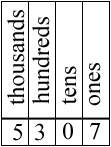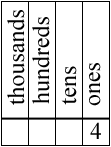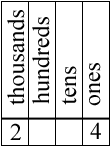SEARCH HOMEMath Central Quandaries & QueriesQuestion from Ivana, a parent: the digit in the ones place is a hand with out a thumb(4) 2) the digit in the thousands place is 2 less than the digit in the one's place. (4-2= 3) the digit in the ten's place is the sum of 2 and 6 (2+6= 4) the digit in the hundreds place is what you get when you get when you subtract 5 from the digit in the ten's place (ten's-5= what is the number?Ivana,

Consider the number 5307. It has a 5 in the thousands place, a 3 in the hundreds place, a 0 in the tens place and a 7 in the ones place.What about your number? It has a 4 in the ones place.The digit in the thousands place is 2 less than the digit in the ones place. That is 4 - 2 = 2 so the digit in the thousands place is 2.Can you complete it now?

PennyMath Central is supported by the University of Regina and The Pacific Institute for the Mathematical Sciences.